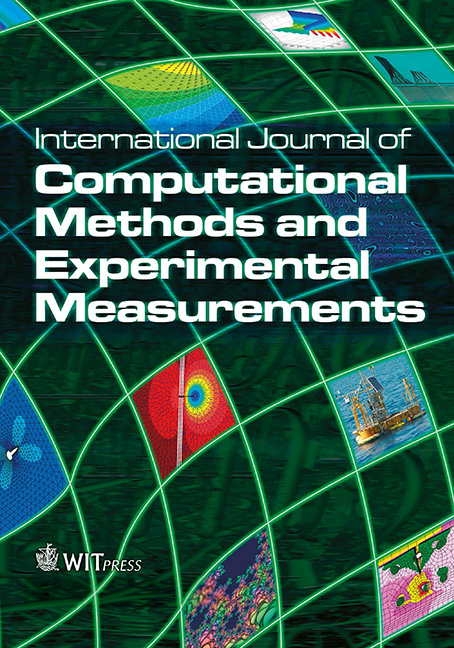WIT Press

The theoretical and experimental study of deterministic chaos phenomena in a process of liquid droplets formation

Price

Free (open access)Volume

Volume 4 (2016), Issue 1

Pages

10

Page Range

13 - 23

Paper DOI

10.2495/CMEM-V4-N1-13-23

WIT Press

Author(s)

M. R. RZASA & B. DOBROWOLSKI

Abstract

The subject matter of this paper involves the examination of the process of liquid drop formation at the outlet of a nozzle. A theoretical model of the formation of liquid droplet has been developed. The model is based on a recursive equation. Solution of this equation is obtained by the solution set, which is similar to the process of liquid droplets formation. The objective is to demonstrate that a recursive theoretical model can reproduce the chaotic behavior of physical phenomena, which is the formation of liquid droplets. In this paper, it is the assessment of the non-stationary characteristics in the process of drop formation. The potential for describing it in terms of principles familiar from deterministic chaos is presented. The paper contains the results of experiments which indicate that chaotic phenomena occur during the formation of liquid drops. The results of the research are elaborated and presented in the form of attractors and power spectrum diagrams. The ranges of the parameters are determined for which the time intervals between the successive drops tend to be non-stationary. Their presentation in the phase space has revealed the occurrence of chaotic phenomena. It was concluded that numerical calculations and the results gained from experiment are comparable. Consequently, it was indicated that relatively simple mathematical model can be used for simulation of the states of actual physical processes.

Keywords

attractors, chaos, drop formation, mathematical models.